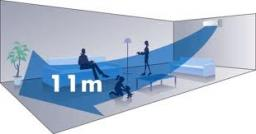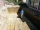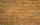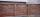# Air

Room is 35.6 m long, 19.6 dm wide and 591 cm high. How many people can simultaneously be in this room if for hygiene reasons is calculated 5000 dm3 of air per person?

n =  82

### Step-by-step explanation:Did you find an error or inaccuracy? Feel free to write us. Thank you!Tips to related online calculators
Do you know the volume and unit volume, and want to convert volume units?
Do you want to round the number?

#### You need to know the following knowledge to solve this word math problem:

We encourage you to watch this tutorial video on this math problem:

## Related math problems and questions:

• Living roomHow many people can live in a room with dimensions: a = 4m b = 5m c = 2.5m if one person needs 15m cubic space (i. E. Air . .. )?
• The cuboidThe cuboid is full to the brim with water. The external dimensions are 95 cm, 120 cm, and 60 cm. The thickness of all walls and the bottom is 5 cm. How many liters of water fit into the cuboid?
• The aquariumThe aquarium is 120 cm long, 70 cm wide and 96 cm high. How high does the water reach if they filled 168l of water into the aquarium?
• Four prismsQuestion No. 1: The prism has the dimensions a = 2.5 cm, b = 100 mm, c = 12 cm. What is its volume? a) 3000 cm2 b) 300 cm2 c) 3000 cm3 d) 300 cm3 Question No.2: The prism base is a rhombus with a side length of 30 cm and a height of 27 cm. The height of t
• SnowSnow fell overnight layer of thickness 19 cm. In the morning I had to clear a path 69 m long and one meter wide. How many cubic meters of snow I clear? How many kilos was it? (1 m3 fresh snow weighs 350 kg)
• The excavationThe excavation for the city sewer was 38 m long, 2.2 m wide, and 3 m deep. a) How many cubic meters of soil were dredged b) How many journeys had to be made by one car when removing the soil if they loaded an average of 4.5 m of cubic soil on each journey
• Brick wallWhat is the weight of a solid brick wall that is 30 cm wide, 4 m long and 2 m high? The density of the brick is 1500 kg per cubic meter.
• Cuboid wallsCalculate the cuboid volume if its different walls have an area of 195cm², 135cm², and 117cm².
• Roof repairTo repair the roof, we need 15 pieces of boards 6 m long, 15 cm wide and 25 mm thick. How many euros will we pay for all boards if 1 m3 of boards costs 500 euros?
• PoolThe swimming pool is 10 m wide and 8 m long, and 153 cm deep. How many hectoliters of water are in it if the water is 30 cm below its upper edge?
• The wallWe have to build a cuboid wall with dimensions base 30 cm and 45 cm and height 3.25 meters. Calculate how many we need bricks if we spend 400 pieces of bricks to 1 m3 of wall?
• BricksHow many bricks are needed for a wall 14.5 m long, 2 m high, and 45 cm thick, if 285 bricks are needed for one m3 wall?
• Third dimensionCalculate the third dimension of the cuboid: a) V = 224 m3, a = 7 m, b = 4 m b) V = 216 dm3, a = 9 dm, c = 4 dm
• Wood lumberWooden lumber is 4 m long and has a cross section square with side 15 cm. Calculate: a) the volume of lumber b) the weight of the lumber if 1 m3 weighs 790 kg
• CanisterGasoline is stored in a cuboid canister having dimensions 44.5 cm, 30 cm, 16 cm. What is the total weight of a full canister when one cubic meter of gasoline weighs 710 kg and the weight of empty canister is 1.5 kg?
• Water tank300hl of water was filled into the tank 12 m long and 6 m wide. How high does it reach?
• Brick wallGarden 70 m long and 48 m wide should surround with wall 2.1 meters high and 30 cm thick. Wall will be built on the garden ground. How many will we need bricks if to 1 m³ is required approximately 300 bricks?